2022 NL MVP Odds
+250
2.5 to 1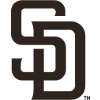Manny Machado
20.2% implied probability

+500
5 to 1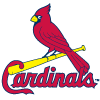Nolan Arenado
11.8% implied probability

+800
8 to 1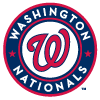Juan Soto
7.8% implied probability

+900
9 to 1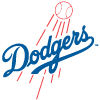Freddie Freeman
7.1% implied probability

+1000
10 to 1Bryce Harper
6.4% implied probability

+1300
13 to 1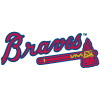Ronald Acuna
5.0% implied probability

+1800
18 to 1Mookie Betts
3.7% implied probability

+2500
25 to 1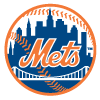Francisco Lindor
2.7% implied probability

+2500
25 to 1Pete Alonso
2.7% implied probability

+3000
30 to 1Trea Turner
2.3% implied probability

+3500
35 to 1Matt Olson
2.0% implied probability

+3500
35 to 1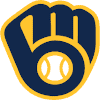Christian Yelich
2.0% implied probability

+4500
45 to 1Austin Riley
1.5% implied probability

+5000
50 to 1Ozzie Albies
1.4% implied probability

+5000
50 to 1Paul Goldschmidt
1.4% implied probability

+5000
50 to 1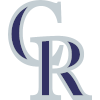C.J. Cron
1.4% implied probability

+5000
50 to 1Willy Adames
1.4% implied probability

+5500
55 to 1Nick Castellanos
1.3% implied probability

+6000
60 to 1Fernando Tatis
1.2% implied probability

+8000
80 to 1Starling Marte
0.9% implied probability

+9000
90 to 1Walker Buehler
0.8% implied probability

No changes have been recorded yet.
+10000
100 to 1Cody Bellinger
0.7% implied probability

+10000
100 to 1Kyle Schwarber
0.7% implied probability

+10000
100 to 1Rhys Hoskins
0.7% implied probability

+10000
100 to 1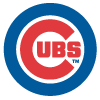Seiya Suzuki
0.7% implied probability

+10000
100 to 1Tyler O'Neill
0.7% implied probability

+10000
100 to 1Max Muncy
0.7% implied probability

+10000
100 to 1Kris Bryant
0.7% implied probability

+10000
100 to 1Marcell Ozuna
0.7% implied probability

+10000
100 to 1J.T. Realmuto
0.7% implied probability

+10000
100 to 1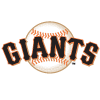Brandon Crawford
0.7% implied probability

+10000
100 to 1Max Scherzer
0.7% implied probability

+15000
150 to 1Jeff McNeil
0.5% implied probability

+15000
150 to 1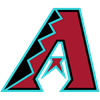Ketel Marte
0.5% implied probability

+15000
150 to 1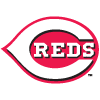Mike Moustakas
0.5% implied probability

+15000
150 to 1Dylan Carlson
0.5% implied probability

+15000
150 to 1Justin Turner
0.5% implied probability

+15000
150 to 1Jonathan India
0.5% implied probability

+15000
150 to 1Charlie Blackmon
0.5% implied probability

+15000
150 to 1Frank Schwindel
0.5% implied probability

+15000
150 to 1Jake Cronenworth
0.5% implied probability

+15000
150 to 1Brandon Belt
0.5% implied probability

+15000
150 to 1Bryan Reynolds
0.5% implied probability

+15000
150 to 1Jean Segura
0.5% implied probability

+15000
150 to 1Paul DeJong
0.5% implied probability

+15000
150 to 1Josh Bell
0.5% implied probability

+15000
150 to 1Jacob deGrom
0.5% implied probability

+15000
150 to 1Joey Votto
0.5% implied probability